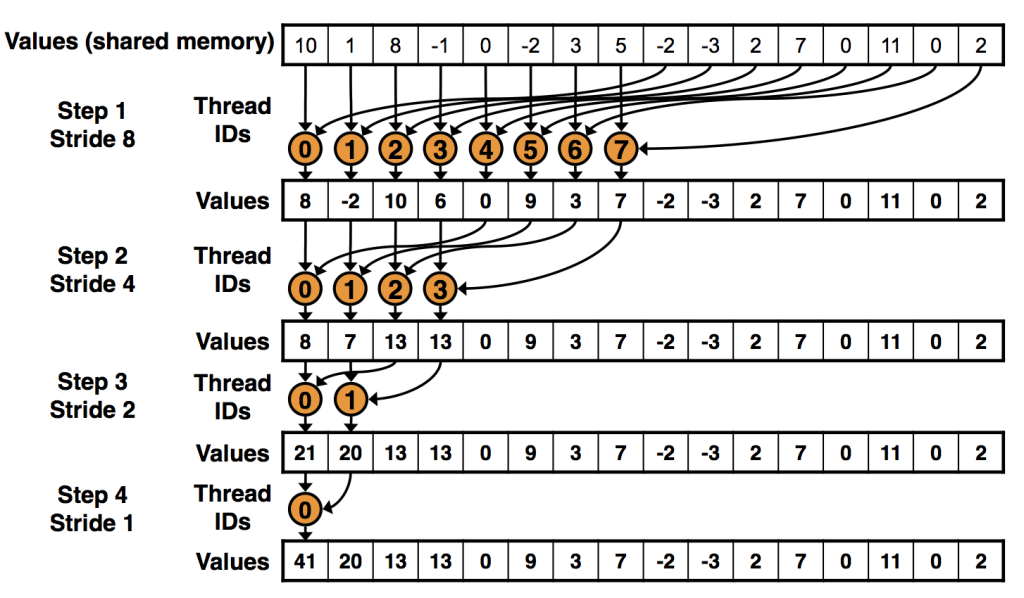# Parallel programming with opencl and python: parallel reduce

Once you know how to use python to run opencl kernels on your device (read Part I and Part II of this series) you need to start thinking about the programming patterns you will use. While many tasks are inherently parallel (like calculating the value of a function for N different values) and you can just straightforwardly run N copies on your processors, most interesting tasks involve dependencies in the data. For instance if you want to simply sum N numbers in the simplest possible way, the thread doing the summing needs to know about all N numbers, so you can only run one thread, leaving most of your cores unused. So what we need to come up with are clever ways to decompose the problem into individual parts which can be run in parallel, and then combine them all in the end.

This is the strong point of Intro to Parallel Programming, available free. If you really want to learn about this topic in depth you should watch the whole course. Here I will only show how I implemented some of the algorithms discussed there in OpenCL (the course is in CUDA). I’ll discuss three algorithms: reduce, scan and histogram. They show how you can use some properties of mathematical operators to decompose a long operation into a series of many small, independent operations.

Reduce is the simplest of the three. Let’s say you have to sum N numbers. On a serial computer, you’d create a temporary variable and add the value of each number to it in turn. It appears there is not much to parallelize here. The key is that addition is a binary associative operator. That means that $a + b + c + d = (a+b) + (c+d)$. So if I have two cores I can add the first two numbers on one, the last two on the other, and then sum those two intermediate results. You can convince yourself that we only need $\mathcal{O}(\log N)$ steps if we have enough parallel processing units; as opposed to $\mathcal{O}(N)$ steps in the serial case. A reduction kernel in OpenCL is straightforward, assuming N is smaller than the number of cores in a single compute unit:

__kernel void reduce(__global float *a,
__global float *r,
__local float *b)
{
uint gid = get_global_id(0);
uint wid = get_group_id(0);
uint lid = get_local_id(0);
uint gs = get_local_size(0);

b[lid] = a[gid];
barrier(CLK_LOCAL_MEM_FENCE);

for(uint s = gs/2; s &gt; 0; s &gt;&gt;= 1) {
if(lid &lt; s) {
b[lid] += b[lid+s];
}
barrier(CLK_LOCAL_MEM_FENCE);
}
if(lid == 0) r[wid] = b[lid];
}


Full code here. First we copy the numbers into shared memory. To make sure we always access memory in a contiguous fashion, we then take the first N/2 numbers and add to them the other N/2, so now we have N/2 numbers to add up. Then we take half of those and add the next half, until there is only one number remaining. That’s the one we copy back to main memory.Reduction stages. Image from nvidia slides.

If we have N bigger than the number of cores in a single unit, we need to call the kernel multiple times. Each core will compute its final answer, and then we run reduce again on that array of answers until we have our final number. In the python code I linked to, you can see how we enqueue the kernel twice, but with fewer threads the second time:

'''run kernels the first time for all N numbers, this will result
in N/n_threads numbers left to sum'''
evt = prg.reduce(queue, (N,), (n_threads,), a_buf, r_buf, loc_buf)
evt.wait()
print evt.profile.end - evt.profile.start